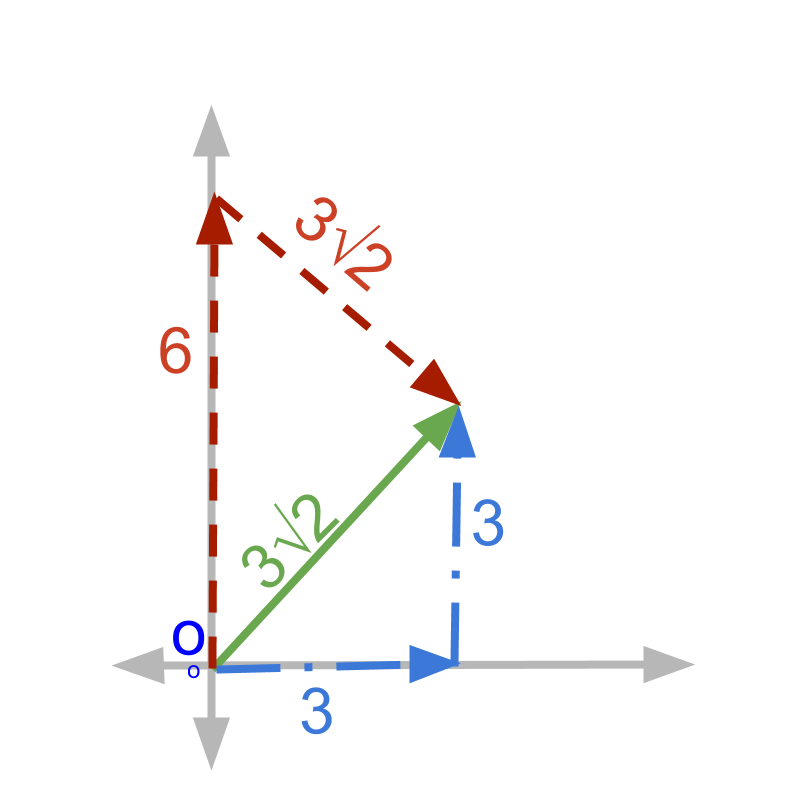maths > vector-algebra

Vector as sum of vectors

what you'll learn...

Overview

Vector as Sum of Vectors

»  numerical expression in place of numbers
→  $2$$2$ can be represented with $4-2$$4 - 2$ or $\frac{34}{17}$$\frac{34}{17}$
All these represent the same number.

»  Vectors as Linear combination of vectors→  $3\sqrt{2}$$3 \sqrt{2}$ at ${45}^{\circ }$${45}^{\circ}$ angle
→  $3$$3$ at ${0}^{\circ }$${0}^{\circ}$ + $3$$3$ at ${90}^{\circ }$${90}^{\circ}$
→  $6$$6$ at ${90}^{\circ }$${90}^{\circ}$ + $3\sqrt{2}$$3 \sqrt{2}$ at $-{45}^{\circ }$$- {45}^{\circ}$
All these represent the same vector.

linear combination

We studied numerical expressions. The following expressions represent the same quantity.
$\quad \quad 5$
$\quad \quad 2 + 3$
$\quad \quad 6 - 1$

A quantity can be equivalently represented with severals forms of numerical expressions.Three persons, starting from a common point, are walking
•  Person A walks at an angle ${45}^{\circ }$${45}^{\circ}$ for $3×\sqrt{2}$$3 \times \sqrt{2}$ meter
•  Person B walks $3$$3$ meter at an angle ${0}^{\circ }$${0}^{\circ}$ and then 3 meter at an angle ${90}^{\circ }$${90}^{\circ}$
•  Person C walks $6$$6$ meter at an angle ${90}^{\circ }$${90}^{\circ}$ and then $3 ×\sqrt{2}$ meter at an angle $-{45}^{\circ }$

To walk back to the starting point, all three has to walk 'same distance in the same direction'.

The three vectors given above are effectively the same vector in three different forms. A vector quantity can be equivalently represented by combination of several vectors.

The walk of Person A (at an angle ${45}^{\circ }$${45}^{\circ}$ for $3×\sqrt{2}$$3 \times \sqrt{2}$ meter) is one vector quantity $3\sqrt{2}$$3 \sqrt{2}$ at angle ${45}^{\circ }$${45}^{\circ}$

The walk of Person B ()$3$$3$ meter at an angle ${0}^{\circ }$${0}^{\circ}$ and then 3 meter at an angle ${90}^{\circ }$${90}^{\circ}$) is sum of two vector quantities $3$$3$ at ${0}^{\circ }$${0}^{\circ}$ and $3$$3$ at ${90}^{\circ }$${90}^{\circ}$.

Note that person A’s and person B’s walks are specified by one vector quantity and two vector quantities. Still, they are at the same distance and direction from the starting point. Effectively, their return to the starting point can be specified by one identical vector quantity.

To understand this, Consider the example in scalar quantities. Person A has $3$$3$ apples in a basket. Person B has $2$$2$ apples in one hand and $1$$1$ apple in another hand. Both has the same number of apples, which is understood as $2+1 = 3$.

Given vectors
$\stackrel{\to }{x}=2i+3j+4k$$\vec{x} = 2 i + 3 j + 4 k$

$\stackrel{\to }{y}=-1i-1j-2k$$\vec{y} = - 1 i - 1 j - 2 k$

$\stackrel{\to }{z}=i+2j+2k$$\vec{z} = i + 2 j + 2 k$
Note that $\stackrel{\to }{x}+\stackrel{\to }{y}=i+2j+2k$$\vec{x} + \vec{y} = i + 2 j + 2 k$ is same as $\stackrel{\to }{z}$$\vec{z}$. So $\stackrel{\to }{z}$$\vec{z}$ can be equivalently given as $\stackrel{\to }{x}+\stackrel{\to }{y}$$\vec{x} + \vec{y}$. Reiterating that A vector can be equivalently given as a sum of vectors.

summary

Linear Combination of Vectors: A vector can be equivalently represented as sum of vectors.

A vector can be equivalently given as the sum of vectors.

Outline